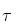Courses

# Notes | EduRev

## NEET Revision Notes

Created by: Learners Habitat

## JEE : Notes | EduRev

The document Notes | EduRev is a part of the JEE Course NEET Revision Notes.
All you need of JEE at this link: JEE

Question 1: One end of an aluminum wire whose diameter is 2.5 mm is welded to one end of a copper wire whose diameter is 1.8 mm. The composite wire carries a steady current i of 17 mA.
(a) What is the current density in each wire?
(b) What is the drift speed of the conduction electrons in the copper wire? Assume that, on the average, each copper atom contributes one conduction electron.
Solution:
(a) We may take the current density as constant with in each wire (except near the junction, where the diameter changes). The cross-sectional area A of the aluminum wire is
AAl = π(d/2)2
= π/4 (2.5×10-3 m)2
= 4.91×10-6m2
And the current density is given by the equation J = i/A,
JAl = i/A
= i/AAl
= (17×10-3 A)/(4.91×10-6 m2)
= 3.5×103 A/m2
As you can verify, the cross-sectional area of the copper wire is 2.54×10-6 m2, so
JCu = i/ACu
= (17×10-3 A)/(2.54×10-6 m2)
=6.7×103A/m2
From the above observation we conclude that, the current density in aluminum wire would be 3.5×103 A/m2 and copper wire would be 6.7×103A/m2.
(b) We can find the drift speed from equation J = (ne)vd if we first find n, the number of electrons per     unit volume. With the given assumption of about one conduction electron per atom, n is the same     as the number of atoms per unit volume and can be found from n/NA = ρ/M or [atoms/m3]/[atoms/mol] = [mass/m3]/[mass/mol].
Here ρ is the density of copper, NA is Avogadro’s number, and M is the molar mass of copper. Thus
n= NAρ/M
= (6.02×1023 mol-1) (9.0×103 kg/m3)/(64×10-3 kg/mol
=8.47×1028 electrons/m3
We then have from equation J = (ne)vd,
v= [6.7×103A/m2]/[(8.47×1028 electrons/m3)(1.6×10-19 C/electron)]
= 4.9×10-7m/s = 1.8 mm/h
From the above observation we conclude that, the drift speed of the conduction electrons in the copper wire would be 1.8 mm/h.

Question 2: A rectangular block of iron has dimensions 1.2 cm×1.2 cm×15 cm.
(a) What is the resistance of the block measured between the two square ends?
(b) What is the resistance between two opposite rectangular faces?
Solution:
(a) The resistivity of iron at room temperature is 9.68×10-8 Ω.m. The area of a square end is (1.2×10-2m)2, or 1.44×10-4 m2. From equation R = ρL/A,
R= ρL/A
= [(9.68×10-8 Ω.m)(0.15 m)]/ [1.44×10-4 m2]
=1.0×10-4 Ω
= 100μΩ
Therefore, the resistance of the block measured between the two square ends would be 100μΩ.
(b) The area of a rectangular face is (1.2×10-2m) (0.15 m), or 1.80×10-3 m2. From equation R = ρL/A,
R = ρL/A
= [(9.68×10-8 Ω.m)(1.2×10-2m)]/ [1.80×10-3 m2]
= 6.5×10-7Ω
= 0.65 μΩ
From the above observation we conclude that, the resistance between two opposite rectangular faces would be 0.65 μΩ.

Question 3:   (IIT-JEE):-
(a) What is the mean free path time τ between collisions for the conduction electrons in copper?
(b) What is the mean free path λ for these collisions? Assume an effective speed veff of 1.6×106 m/s.
Solution:
(a) We know that,
ρ=m/ne2The number of conduction electrons per unit volume (n) in copper will be,
n/NA = ρ/M or [atoms/m3]/[atoms/mol] = [mass/m3]/[mass/mol]
Here ρ is the density of copper, NA is Avogadro’s number, and M is the molar mass of copper. Thus
n = NAρ/M
= (6.02×1023 mol-1) (9.0×103 kg/m3)/(64×10-3 kg/mol)
=8.47×1028 electrons/m3
The value of ρ for copper is 1.69×10-8 Ω.m.
So the denominator of the above equation will be,
ne2= (8.47×1028 electrons/m3) (1.6×10-19C)2(1.69×10-8 Ω.m)
= 3.66×10-17C2. Ω/m2
= 3.66×10-17 kg/s
Here we converted units as,
C2. Ω/m2 = C2. V/m2.A = (C2.J/C)/(m2.C/s) = (kg.m2/s2)/(m2/s) = kg/s
For the mean free time we then have,= (9.1×10-31kg)/(3.66×10-17 kg/s)
= 2.5×10-14s
Therefore, the mean free path time τ between collisions for the conduction electrons in copper would be 2.5×10-14s.
(b) We defined the mean free path as being the average distance traversed by a particle between collisions. Here the time between collisions of a free electron is τ and the speed of the electron is veff .
So, λ =veff
= (2.5×10-14s) (1.6×106 m/s)
= 4.0×10-8 m = 40 nm
From the above observation, we conclude that the mean free path λ for these collisions would be 40 nm. This is about 150 times the distance between nearest- neighbor atoms in a copper lattice.

Offer running on EduRev: Apply code STAYHOME200 to get INR 200 off on our premium plan EduRev Infinity!

291 docs

,

,

,

,

,

,

,

,

,

,

,

,

,

,

,

,

,

,

,

,

,

;